Advertisement Remove all ads

# Selina solutions for Concise Physics Class 10 ICSE chapter 3 - Machines [Latest edition]

#### Chapters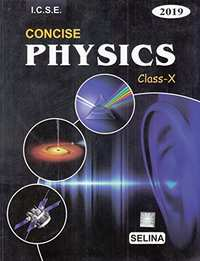Advertisement Remove all ads
Advertisement Remove all ads
Advertisement Remove all ads

## Chapter 3: Machines

Exercise 3 (A)Exercise 3 (B)Others
Advertisement Remove all ads
Exercise 3 (A) [Pages 57 - 58]

### Selina solutions for Concise Physics Class 10 ICSE Chapter 3 MachinesExercise 3 (A) [Pages 57 - 58]

Exercise 3 (A) | Q 1.1 | Page 57

What do you understand by a simple machine?

Exercise 3 (A) | Q 1.2 | Page 57

Stat the principle of an ideal machine.

Exercise 3 (A) | Q 2 | Page 57

State four ways in which machines are useful to us?

Exercise 3 (A) | Q 3.1 | Page 57

Name a machine to multiply force ?

Exercise 3 (A) | Q 3.2 | Page 57

Name a machine to change the point of application of force ?

Exercise 3 (A) | Q 3.3 | Page 57

Name a machine to change the direction of force ?

Exercise 3 (A) | Q 3.4 | Page 57

Name a machine to obtain gain in speed ?

Exercise 3 (A) | Q 4 | Page 57

What is the purpose of a jack in lifting a car by it?

Exercise 3 (A) | Q 5.1 | Page 57

What do you understand by an ideal machine?

Exercise 3 (A) | Q 5.2 | Page 57

How Does Ideal Machine Differ from a Practical Machine ?

Exercise 3 (A) | Q 6 | Page 57

Explain the term mechanical advantage. State its unit.

Exercise 3 (A) | Q 7 | Page 57

Define the term velocity ratio, state its unit.

Exercise 3 (A) | Q 8 | Page 57

How is mechanical advantage related to the velocity ratio for an ideal machine?

Exercise 3 (A) | Q 9.1 | Page 57

Define the term efficiency of a machine.

Exercise 3 (A) | Q 9.2 | Page 57

Why is a machine not 100% efficient?

Exercise 3 (A) | Q 10.1 | Page 57

When does a machine act as a force multiplier ?

Exercise 3 (A) | Q 10.2 | Page 57

When does a machine act as a speed multiplier ?

Exercise 3 (A) | Q 10.3 | Page 57

Can a machine act as a force multiplier and a speed multiplier simultaneously?

Exercise 3 (A) | Q 11 | Page 57

A machine works as a
(i) force multiplier,
(ii) speed multiplier.
In each case state whether the velocity ratio is more than or less than 1.

Exercise 3 (A) | Q 12 | Page 57

(i) State the relationship between mechanical advantage, velocity ratio and efficiency.

(ii) Name the term that will not change for a machine of a given design.

Exercise 3 (A) | Q 13 | Page 57

Derive the relationship between mechanical advantage, velocity ratio and efficiency of a machine.

Exercise 3 (A) | Q 14 | Page 57

How is the mechanical advantage related with the velocity ratio for an actual machine? State whether the efficiency of such a machine is equal to 1, less than 1 or more than 1.

Exercise 3 (A) | Q 15 | Page 57

State reason why is mechanical advantage less than the velocity ratio for an actual machine.

Exercise 3 (A) | Q 16.1 | Page 57

What is a lever ?

Exercise 3 (A) | Q 16.2 | Page 57

State the principle of a lever ?

Exercise 3 (A) | Q 17 | Page 57

Write down a relation expressing the mechanical advantage of a lever.

Exercise 3 (A) | Q 18 | Page 57

Name the three classes of levers and distinguish between them. Give two examples of each class.

Exercise 3 (A) | Q 19.1 | Page 57

Give one example of a class I lever where mechanical advantage is more than one ?

Exercise 3 (A) | Q 19.2 | Page 57

Give one example of a class I lever where mechanical advantage is  less than one ?

Exercise 3 (A) | Q 20 | Page 57

What is the use of the lever if its mechanical advantage is  (a) more than 1, (b) equal to 1, and (c) less than 1 ?

Exercise 3 (A) | Q 21 | Page 57

A pair of scissors and a pair of pliers both belongs to the same class of levers. Name the class of lever. Which one has the mechanical advantage less than 1?

Exercise 3 (A) | Q 22 | Page 57

Explain why scissors for cutting cloth may have blades longer than the handles, but shears for cutting metals have short blades and long handles.

Exercise 3 (A) | Q 23 | Page 57

shows a uniform metre scale of weight W supported on a fulcrum at the 60 cm mark by applying the effort E at the 90 cm mark.
(a) state with reason whether the weight W of the scale is greater than, less than or equal to the effort E.

(b) Find the mechanical advantage in an ideal case.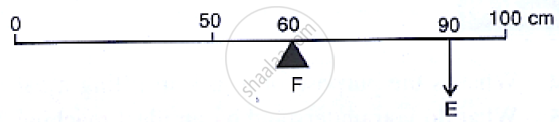Exercise 3 (A) | Q 24 | Page 57

Which type of lever has a mechanical advantage always more than one? Give one example. What change can be made in this lever to increase its mechanical advantage?

Exercise 3 (A) | Q 25 | Page 58

Draw a diagram of a lever which is always used as a force multiplier. How is the effort arm related to the load arm in such a lever?

Exercise 3 (A) | Q 26 | Page 58

Explain why the mechanical advantage of a Class II type of lever is always more than 1.

Exercise 3 (A) | Q 27 | Page 58

Draw a labelled diagram of a class II lever. Give one example of such a lever.

Exercise 3 (A) | Q 28.1 | Page 58

Shows a lemon crusher.

In the diagram, mark the position of the directions of load L and effort E.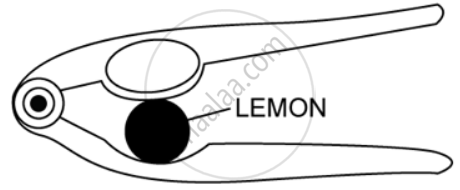Exercise 3 (A) | Q 28.2 | Page 58

Shows a lemon crusher.  Name the class of lever.

Exercise 3 (A) | Q 29 | Page 58

The diagram below shows a rod lifting a stone.

(a) Mark position of fulcrum F and draw arrows to show the directions of load L and effort E.

(b) What class of lever is the rod?

(c) Give one more example of the same class of lever stated in part (b).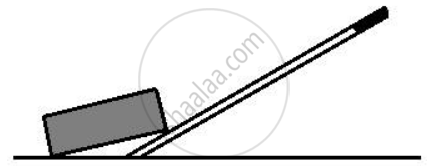Exercise 3 (A) | Q 30 | Page 58

State the kind of lever which always has the mechanical advantage less than 1. Draw a labelled diagram of such lever.

Exercise 3 (A) | Q 31 | Page 58

Explain why the mechanical advantage of the class III type of lever is always less than 1.

Exercise 3 (A) | Q 32 | Page 58

Class III levers have mechanical advantage less than one. Why are they then used?

Exercise 3 (A) | Q 33 | Page 58

Draw a labelled sketch of a class III lever. Give one example of this kind of lever.

Exercise 3 (A) | Q 34.1 | Page 58

State the class of levers and the relative positions of load (L) effort (E) and fulcrum (F) in a bottle opener?

Exercise 3 (A) | Q 34.2 | Page 58

State the class of levers and the relative positions of load (L) effort (E) and fulcrum (F) in sugar tongs.

Exercise 3 (A) | Q 35.1 | Page 58

Draw Diagrams to illustrate the position of fulcrum load and effort, in the following :

A seesaw .

Exercise 3 (A) | Q 35.2 | Page 58

Draw Diagrams to illustrate the position of fulcrum load and effort, in the following:

A common balance .

Exercise 3 (A) | Q 35.3 | Page 58

Draw Diagrams to illustrate the position of fulcrum load and effort, in the following:

A nut cracker .

Exercise 3 (A) | Q 35.4 | Page 58

Draw Diagrams to illustrate the position of fulcrum load and effort, in the following:

Forceps .

Exercise 3 (A) | Q 36.1 | Page 58

Classify the following into lever as class I, class II or class III of  a door ?

Exercise 3 (A) | Q 36.2 | Page 58

Classify the following into lever as class I, class II or class III  of a catapult ?

Exercise 3 (A) | Q 36.3 | Page 58

Classify the following into lever as class I, class II or class III of a wheel barrow ?

Exercise 3 (A) | Q 36.4 | Page 58

Classify the following into lever as class I, class II or class III of a fishing rod ?

Exercise 3 (A) | Q 37.1 | Page 58

What type of lever is formed by the human body while raising a load on the palm ?

Exercise 3 (A) | Q 37.2 | Page 58

What type of lever is formed by the human body while raising the weight of body on toes?

Exercise 3 (A) | Q 38 | Page 58

Indicate the positions of load, effort and fulcrum in the forearm shown below in Fig 3.15 Name class of lever.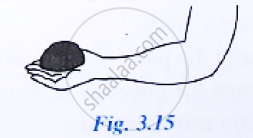Exercise 3 (A) | Q 39 | Page 58

Give example of each class of lever in a human body?

Exercise 3 (A) | Q 40.1 | Page 58

Complete the following sentence :

Mechanical advantage = ___________ × velocity ratio

Exercise 3 (A) | Q 40.2 | Page 58

Complete the Following Sentence :

In class II lever, effort arm is __________ than the load arm.

Exercise 3 (A) | Q 40.3 | Page 58

Complete the following sentence :

A scissors is a ___________ multiplier.

Exercise 3 (A) [Page 58]

### Selina solutions for Concise Physics Class 10 ICSE Chapter 3 MachinesExercise 3 (A) [Page 58]

Exercise 3 (A) | Q 1 | Page 58

Mechanical advantage (M.A), load (L) and effort (E) are related as :

• M.A. = L x E

• M.A. x E = L

• E = M.A. x L

• None of these

Exercise 3 (A) | Q 2 | Page 58

The correct relationship between the mechanical advantage (M.A), the velocity ratio (V.R) and the efficiency (n) is :

• M.A. = η x V.R

• V.R. = η x M.A.

• η = M.A. x V.R.

• None of these

Exercise 3 (A) | Q 3 | Page 58

State the incorrect statement:

• A machine always has the efficiency less than 100%

• The mechanical advantage of a machine can be less than 1.

• A machine can be used as speed multiplier.

• A machine can have the mechanical advantage greater than the velocity ratio.

Exercise 3 (A) | Q 4 | Page 58

The lever for which the mechanical advantage is less than 1 has :

• Fulcrum at mid-point between load and effort.

• Load between effort and fulcrum.

• Effort between fulcrum and load.

• Load and effort acting at the same point.

Exercise 3 (A) | Q 5 | Page 58

Class II levers are designed to have:

•  M.A. = V.R.

• M.A. > V.R.

• M.A. > 1

• M.A. < 1

Exercise 3 (A) [Pages 59 - 60]

### Selina solutions for Concise Physics Class 10 ICSE Chapter 3 MachinesExercise 3 (A) [Pages 59 - 60]

Exercise 3 (A) | Q 1 | Page 59

A crowbar of length 120 cm has its fulcrum situated at a distance of 20 cm from the load. Calculate the mechanical advantage of the crowbar.

Exercise 3 (A) | Q 2 | Page 59

A pair of scissors has its blades 15 cm long, while its handles are 7.5 cm long. What is its mechanical advantage?

Exercise 3 (A) | Q 3 | Page 59

A force of 5 kgf is required to cut a metal sheet. A shears used for cutting the metal sheet has its blades 5 cm long, while its handles is 10 cm long. What effort is needed to cut the sheet?

Exercise 3 (A) | Q 4 | Page 59

Fig 3.16 below shows a lever in use.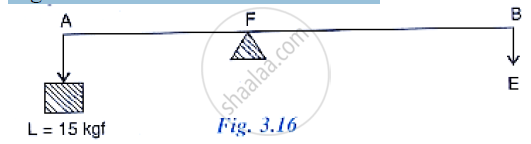(a) To which class of lever does it belong?
(b) If AB = 1m, AF = 0.4 m, find its mechanical advantage,
(c) calculate the value of E.

Exercise 3 (A) | Q 5 | Page 59

A man uses a crowbar of length 1.5 m to raise a load of 75 kgf by putting a sharp edge below the bar at a distance 1 m from his hand. Draw a diagram of the arrangement showing the fulcrum (F), load (L) and effort (E) with their directions. State the kind of lever. Calculate: (1) load arm, (2) effort arm, (3) mechanical advantage and (4) the effort needed.

Exercise 3 (A) | Q 6 | Page 59

A pair of scissors is used to cut a piece of a cloth by keeping it at a distance 8.0 cm from its rivet and applying an effort of 10 kgf by fingers at a distance 2.0 cm from the rivet. (a) Find : (i) the mechanical advantage of scissors and (ii) the load offered by the cloth (b) How does the pair of scissors act: as a force multiplier or as speed multiplier?

Exercise 3 (A) | Q 7 | Page 59

A 4 m long rod of negligible weight is to be balanced about a point 125 cm from one end. A load of 18 kgf is suspended at a point 60 cm from the support on the shorter arm.
(a) a weight W is placed 250 cm from the support on the longer arm Find W.
(b) If W = 5 kgf, where must it be kept to balance the rod?
(c) To which class of lever does it belong?

Exercise 3 (A) | Q 8.1 | Page 59

A lever of length 9 cm has its load arm 5 cm long and the effort arm is 9 cm long.

To which class does it belong ?

Exercise 3 (A) | Q 8.2 | Page 59

A lever of length 9 cm has its load arm 5 cm long and the effort arm is 9 cm long.

Draw diagram of the lever showing the position of fulcrum F and directions of both the load L and effort E.

Exercise 3 (A) | Q 8.3 | Page 59

A lever of length 9 cm has its load arm 5 cm long and the effort arm is 9 cm long.

What is the mechanical advantage and velocity ratio if the efficiency is 100%?

Exercise 3 (A) | Q 8.4 | Page 59

A lever of length 9 cm has its load arm 5 cm long and the effort arm is 9 cm long.

What will be the mechanical advantage and velocity ratio if the efficiency becomes 50% ?

Exercise 3 (A) | Q 9 | Page 59

Fig 3.17 below shows a lever in use.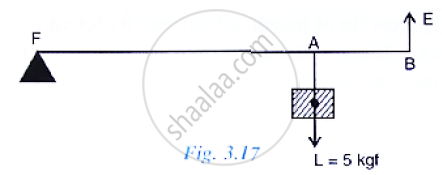(a) To which class of lever does it belong?
(b) If FA = 80 cm, AB = 20 cm, find its mechanical advantage.
(c) Calculate the value of E.

Exercise 3 (A) | Q 10 | Page 59

Fig 3.19 below shows a wheel barrow of mass 15 kg carrying a load of 30 kgf with its centre of gravity at A. The points B and C are the centre of wheel and tip of the handle such that the horizontal distance AB = 20 cm and AC = 40 cm.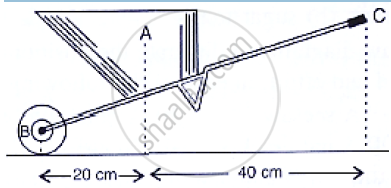Calculate: (i) the load arm, (ii) the effort arm, (iii) the mechanical advantage and (iv) the minimum effort required to keep the leg just off the ground.

Exercise 3 (A) | Q 11 | Page 60

Fig 3.18 below shows the use of a lever.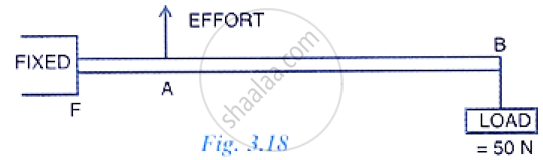(a) State the principle of moments as applied to the above lever.
(b) Give an example of this class of lever.
(c) If FA = 10 cm, AB = 500 cm calculate: (i) the mechanical advantage and (ii) the minimum effort required to lift the load.

Exercise 3 (A) | Q 12 | Page 60

A fire tongs has arms 20 cm long. Its is used to lift a coal of weight 1.5 kgf by applying an effort at a distance 15 cm from the fulcrum. Find: (i) the mechanical advantage of fire tongs and (ii) the effort needed.

Exercise 3 (B) [Pages 66 - 67]

### Selina solutions for Concise Physics Class 10 ICSE Chapter 3 MachinesExercise 3 (B) [Pages 66 - 67]

Exercise 3 (B) | Q 1 | Page 66

What is a single fixed pulley? State its one use.

Exercise 3 (B) | Q 2 | Page 66

What is the ideal mechanical advantage of a single fixed pulley? Can it be used as a force
multiplier?

Exercise 3 (B) | Q 3 | Page 66

Name the pulley which has no gain in mechanical advantage. Explain, why is such a pulley then used?

Exercise 3 (B) | Q 4 | Page 66

What is the velocity ratio of a single fixed pulley?

Exercise 3 (B) | Q 5 | Page 66

In a single fixed pulley, if the effort moves by a distance x downwards, by what height is the load raised upwards?

Exercise 3 (B) | Q 6 | Page 66

What is a single movable pulley? What is its mechanical advantage in the ideal case?

Exercise 3 (B) | Q 7 | Page 66

Name the type of single pulley that can act as a force multiplier. Draw a labelled diagram of the pulley mentioned by you.

Exercise 3 (B) | Q 8 | Page 66

Give two reasons why the efficiency of a single movable pulley system is not 100%?

Exercise 3 (B) | Q 9 | Page 66

In which direction the force need to applied, when a single pulley is used with a mechanical advantage greater than one? How can you change the direction of force applied without altering its mechanical advantage? Draw a labelled diagram of the system.

Exercise 3 (B) | Q 10 | Page 67

What is the velocity ratio of a single movable pulley ? How does the friction in the pulley bearing affect it?

Exercise 3 (B) | Q 11 | Page 67

In a single movable pulley, if the effort moves by a distance x upwards, by what height is the load raised?

Exercise 3 (B) | Q 12 | Page 67

Draw a labelled diagram of an arrangement of two pulleys, one fixed and other movable. In the diagram, mark the directions of al forces acting on it. What is the ideal mechanical advantage of the system? How can it be achieved?

Exercise 3 (B) | Q 13 | Page 67

The diagram below shows a pulley arrangement.
(a) In the diagram, mark the direction of tension on each strand of string.
(b) What is the purpose of the pulley B?

(c) If the tension is T, Deduce the relation between T and E.
(d) What is the velocity ratio of the arrangement?
(e) Assuming that the efficiency of the system is 100%, What is the mechanical advantage?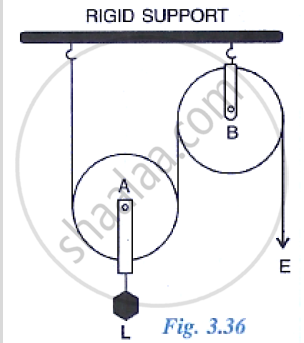Exercise 3 (B) | Q 14 | Page 67

Differentiate between a single fixed pulley and a single movable pulley.

Exercise 3 (B) | Q 15 | Page 67

The Diagram alongside shows an arrangement of three pulleys A, B, and C. The load is marked as L and the effort as E.
(a) Name the Pulleys A, B, and C.
(b) Mark in the diagram the directions of load (L), effort (E) and tension T1 and T2 in the two strings.
(c) How are the magnitudes of L and E related to the tension T1?
(d) Calculate the mechanical advantage and velocity ratio of the arrangement.
(e) What assumptions have you made in parts (c) and (d)?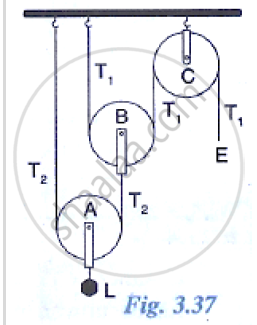Exercise 3 (B) | Q 16 | Page 67

Draw a diagram of combination of three movable pulleys with a fixed pulley showing the directions of load, effort and tension in each strand. Find: (i) mechanical advantage, (ii) Velocity ration and (iii) efficiency of the combination in ideal situation.

Exercise 3 (B) | Q 17 | Page 67

A block and tackle pulley system has a velocity ratio 5. Draw a labelled diagram of this system.  In your diagram, indicate clearly the points of application and the directions of the load and effort.

Exercise 3 (B) | Q 18.1 | Page 67

Give reason for the following:
In a single fixed pulley, the velocity ratio is always more than the mechanical advantage.

Exercise 3 (B) | Q 18.2 | Page 67

Give reason for the following:

The efficiency of a movable pulley is always less than 100%.

Exercise 3 (B) | Q 18.3 | Page 67

Give reason for the following:

In case of a block and tackle arrangement, the mechanical advantage increases with the increase in the number of pulleys.

Q 18.4

Give reason for the following:

The lower block of a block and tackle pulley system must be of negligible weight

Exercise 3 (B) | Q 19.1 | Page 67

Name a machine to multiply force ?

Exercise 3 (B) | Q 19.2 | Page 67

Name a machine which is used to multiply speed ?

Exercise 3 (B) | Q 19.3 | Page 67

Name a machine which is used to change the direction for force applied.

Exercise 3 (B) | Q 20.1 | Page 67

State whether the following statement is true or false:

The velocity ratio of a single fixed pulley is always more than 1.

• True

• False

Exercise 3 (B) | Q 20.2 | Page 67

State whether the following statement is true or false:

The velocity ratio of a single movable pulley is always 2.

• True

• False

Exercise 3 (B) | Q 20.3 | Page 67

State whether the following statement is true or false:

The velocity ratio of a combination of n movable pulleys with a fixed pulley is always 2n.

• True

• False

Exercise 3 (B) | Q 20.4 | Page 67

State whether the following statement is true or false:

The velocity ratio of a block and tackle system is always equal to the number of strands of the tackle supporting the load.

• True

• False

Exercise 3 (B) [Page 68]

### Selina solutions for Concise Physics Class 10 ICSE Chapter 3 MachinesExercise 3 (B) [Page 68]

Exercise 3 (B) | Q 1 | Page 68

A Single fixed pulley is used because it :

• Has a mechanical advantage greater than 1

• Has a velocity ratio less than 1

• Gives 100% efficiency

• Helps to apply the effort in a convenient direction.

Exercise 3 (B) | Q 2 | Page 68

The mechanical advantage of an ideal single movable pulley is

• 1

• 2

• less than 2

• less than 1

Exercise 3 (B) | Q 3 | Page 68

A movable pulley is used as :

• a force multiplier

• a speed multiplier

• a device to change the direction of effort

• an energy multiplier

Exercise 3 (B) [Pages 68 - 69]

### Selina solutions for Concise Physics Class 10 ICSE Chapter 3 MachinesExercise 3 (B) [Pages 68 - 69]

Exercise 3 (B) | Q 1 | Page 68

A Woman draws water from a well using a fixed pulley. The mass of bucket and water together is 6 kg. The force applied by the woman is 70 N. Calculate the mechanical advantage. ( Take g = 10 m s-2)

Exercise 3 (B) | Q 2.1 | Page 68

A fixed pulley is driven by a 100 kg mass falling at a rate of 8.0 m in 4.0s. It lifts a load of 75.0 kgf.

Calculate the power input to the pulley taking the force of gravity on 1 kg as 10 N.

Exercise 3 (B) | Q 2.2 | Page 68

A fixed pulley is driven by a 100 kg mass falling at a rate of 8.0 m in 4.0 s. It lifts a load of 75.0 kgf.

Calculate the efficiency of the pulley.

Exercise 3 (B) | Q 2.3 | Page 68

A fixed pulley is driven by a 100 kg mass falling at a rate of 8.0 m in 4.0 s. It lifts a load of 75.0 kgf.

Calculate : the height to which the load is raised in 4.0 s.

Exercise 3 (B) | Q 3 | Page 68

A single fixed pulley and a movable pulley both are separately used to lift a load of 50 kgf to the same height. Compare the efforts applied.

Exercise 3 (B) | Q 4 | Page 68

In a block and tackle system consisting of 3 pulleys, a load of 75 kgf is raised with an effort of 25 kgf. Find: (i) the mechanical advantage, (ii) velocity ratio and (iii) efficiency.

Exercise 3 (B) | Q 5 | Page 68

A block and tackle system has 5 pulleys. If an effort 0f 1000 N is needed in the downward direction to raise a load of 4500 N, calculate:
(a) the mechanical advantage
(b) the velocity ratio, and
(c) the efficiency of the system

Exercise 3 (B) | Q 6 | Page 68

In following figure , draw a tackle to lift the load by applying the force in the downward direction. Mark the position of load and effort.
(a) If the load is raised by 1 m, through what distance will the effort move?
(b) State how many strands of tackle are supporting the load?
(c) What is the mechanical advantage of the system?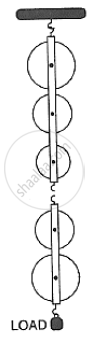Exercise 3 (B) | Q 7 | Page 68

A pulley system has a velocity ratio 3 and an efficiency of 80%. Draw a labelled diagram of this pulley system Calculate:
(a) the mechanical advantage of the system and

(b) the effort required to raise a load of 300 N.

Exercise 3 (B) | Q 8 | Page 68

In following figure , shows a system of four pulleys, The upper two pulleys are fixed and the lower two are movable.
(a) Draw a string around the pulleys. Also show the place and direction in which the effort if applied.
(b) What is the velocity ratio of the system?
(c) How are load and effort of the pulley system related?
(d) What assumption do you make in arriving at your answer in part(c)?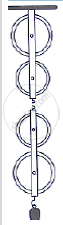Exercise 3 (B) | Q 9 | Page 68

In following figure ,  shows a block and tackle system of pulleys used to lift a load.
(a) How many strands of tackle are supporting the load?
(b) Draw arrows to represent tension in each strand.
(c) What is the mechanical advantage of the system?
(d) When load is pulled up be a distance 1 m, how far does the effort end move?

Exercise 3 (B) | Q 10.1 | Page 69

A block and tackle system has the velocity ratio 3. Draw a labelled diagram of the system indicating the points of application and the directions of load and effort. A man can exert a pull of 200 kgf. What is the maximum load he can raise with this pulley system is its efficiency is 60%?

Exercise 3 (B) | Q 10.2 | Page 69

A block and tackle system has the velocity ratio 3. Draw a labelled diagram of the system indicating the points of application and the directions of load L and effort E. A man can exert a pull of 200 kgf

If the effort end moves a distance 60 cm, what distance does the load move?

Exercise 3 (B) | Q 11 | Page 69

You are given four pulleys and three strings. Draw a neat and labelled diagram to use them so as to obtain a maximum mechanical advantage equal to 8. In you diagram make the directions of load, effort and tension in each strand.
What assumptions have you made to obtain the required mechanical advantage?

## Chapter 3: Machines

Exercise 3 (A)Exercise 3 (B)Others## Selina solutions for Concise Physics Class 10 ICSE chapter 3 - Machines

Selina solutions for Concise Physics Class 10 ICSE chapter 3 (Machines) include all questions with solution and detail explanation. This will clear students doubts about any question and improve application skills while preparing for board exams. The detailed, step-by-step solutions will help you understand the concepts better and clear your confusions, if any. Shaalaa.com has the CISCE Concise Physics Class 10 ICSE solutions in a manner that help students grasp basic concepts better and faster.

Further, we at Shaalaa.com provide such solutions so that students can prepare for written exams. Selina textbook solutions can be a core help for self-study and acts as a perfect self-help guidance for students.

Concepts covered in Concise Physics Class 10 ICSE chapter 3 Machines are Machines as Force Multipliers, Lever, Concept of Pulley System, Principle of Conservation of Energy, Concept of Machines Numercials, Simple Machines.

Using Selina Class 10 solutions Machines exercise by students are an easy way to prepare for the exams, as they involve solutions arranged chapter-wise also page wise. The questions involved in Selina Solutions are important questions that can be asked in the final exam. Maximum students of CISCE Class 10 prefer Selina Textbook Solutions to score more in exam.

Get the free view of chapter 3 Machines Class 10 extra questions for Concise Physics Class 10 ICSE and can use Shaalaa.com to keep it handy for your exam preparation

Advertisement Remove all ads
Share
Notifications

View all notifications

Forgot password?